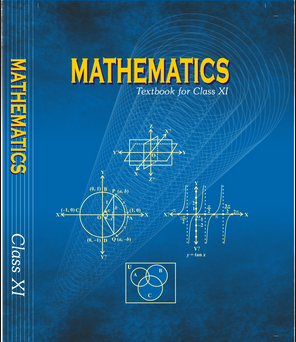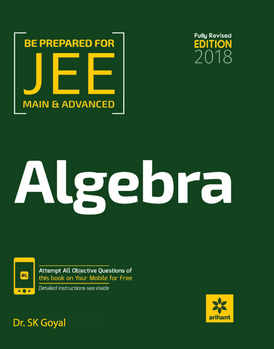# Permutations and combinations   Share

## What is Permutations and combinations

Permutations and Combinations are concerned with determining the number of different ways of arranging and selecting objects out of a given number of objects, without actually listing them.  These are generally referred to as “PnC”. This chapter is all about logic and “counting”. PnC tests your ability to observe the pattern, your mathematical reasoning, and creativity. From the exam point of view,  PnC is one of the important chapters. Concepts of Permutations and combinations are mostly used while solving the problems from probability. The problems of probability are no longer as simple as they used to be in elementary standards. However, if you have a command on Permutations and combinations, the chapter probability will be a piece of cake for you.

## Why Permutation and Combination

Suppose you have a smartphone with a screen lock set as a PIN. The number pad has 10 digits from 0 to 9. The lock can be opened if 4 specific digits are arranged in a particular sequence with no repetition. Somehow, you have forgotten this specific sequence of digits. You remember only the first digit which is 7. In order to open the lock, how many sequences of 3-digits you may have to check with? To answer this question, you may, immediately, start listing all possible arrangements of 9 remaining digits taken 3 at a time. But, this method will be tedious, because the number of possible sequences may be large. (By the way, it would take a maximum of 504 trials to unlock your screen.) Here, in this Chapter, you will study some basic counting techniques which will enable you to answer this question without actually listing 3-digit arrangements. In fact, these techniques will be useful in determining the number of different ways of arranging and selecting objects without actually listing them.

Prepare Online for JEE Main/NEET

Crack JEE 2021 with JEE/NEET Online Preparation Program## Important Topics of Permutation and Combination

1. Fundamental Principle of Counting

2. Permutations as an Arrangement

3. Combinations as Selections

4. P(n,r) and C(n,r)

5. Application of Permutation and Combination

## Overview of the Permutation and Combination

Fundamental Principle of Counting

According to the Addition Principle, if one event can occur in m ways and a second event with no common outcomes can occur in n ways, then one of the first or second events can occur in m + n ways.

If you are shopping for a new computer and you need to decide among 3 desktop computers and 2 laptop computers. Then,  what is the total number of computer options you will have?So as from the figure, you will have a total of 5 options to choose one computer.

According to the Multiplication Principle, if one event can occur in m ways and a second event can occur in n ways after the first event has occurred, then the two events can occur in m × n ways.

Suppose you are choosing an appetizer, an entrée, and a dessert. If there are 2 appetizer options, 3 entrée options, and 2 dessert options on a fixed price dinner menu, there are a total of 12 possible choices of one each as shown in the tree diagramThe possible choices are:

1. soup, chicken, cake

2. soup, chicken, pudding

3. soup, fish, cake

4. soup, fish, pudding

5. soup, steak, cake

6. soup, steak, pudding

You can also find the total number of possible dinners by multiplying.

# of appetizer options × # of entree options × # of dessert options

2               ×              3                ×                  2                = 12

## Permutation

A permutation is an arrangement in a definite order of a number of objects taken some or all at a time.

The total number of permutations of n distinct objects taken r at a time is denoted by P(n,r) or nPr and defined by

Let’s understand this by an example

How many ways can we award a 1st, 2nd and 3rd place prize among five contestants? (Gold / Silver / Bronze)

We’re going to use permutations since the order we hand out these medals matters. Here’s how it breaks down:• Gold medal: 5 choices: A B C D E. Let’s say A wins the Gold.

• Silver medal: 4 choices: B C D E. Let’s say B wins the silver.

• Bronze medal: 3 choices: C D E. Let’s say… C wins the bronze.

We had 5 choices at first, then 4, then 3. The total number of options was

5 × 4 × 3 = 60

This can also be solved using the formula P(n,r).

n = 5 and r = 3

## Combinations

Each of the different group or selection which can be made by taking some or all of a number of objects, irrespective of their arrangement is called combinations. Combinations mean the selection of the object.

The number of selection that can be formed from n different objects  taken r at a time is given by C(n,r) or nCr and defined as

## Difference between Permutation and Combinations

The term permutation refers to several ways of arranging a set of objects in sequential order. Combination implies several ways of choosing items from a large pool of objects, such that their order is irrelevant.

Suppose, you have to find out the total number of possible samples of two out of three objects X, Y, Z. In this question, first of all, you need to understand, whether the question is related to permutation or combination and the only way to find this out is to check whether the order is important or not.

If the order is important, then the question is related to permutation, and possible samples will be, XY, YX, YZ, ZY, ZX, XZ. Where XY is different from YX, YZ is different from ZY and ZX is different from  XZ.

If the order is irrelevant, then the question is related to the combination, and the possible samples will be XY, YZ, and ZX.

## How to Prepare Permutation and Combination

Follow these tips to prepare the chapter permutation and combination created by the subject matter experts:

Understand the use of nPr and nCr:-

When you first prepare for permutation and combination, the two-term you’ll learn arenPr andnCrand initially, you will find that you are using  these two terms wrongly to solve problems. So in the beginning, solve the problems without using them. Slowly by practice, you’ll understand the pattern and the need for these two terms in solving certain questions. And only after that, you’ll learn the usage of these terms perfectly.

Practice Different types of Questions:-
In Permutation and Combination, you can form an infinite number and different type of questions and still cannot be confident that you will be able to solve all questions of this topic. So to be able to solve the maximum number of questions you need to practice a lot, that’s the key. Solve the variety of questions and first try to understand the question. Think about the concept that can be used to solve this question. And don’t jump directly to the solution after just reading the question. In JEE Main questions from this topic won’t be too exaggerated and will test your basics. Make sure you practice the ball-box and other related problems, as many questions previously asked previously in JEE Main revolve around this concept.

## Best Books For Preparation of Permutation and Combination:-

First, finish all the concept, example and question given in NCERT Maths Book. You must thorough with the theory of NCERT. Then you can refer to the book Cengage Mathematics Algebra. PnC is explained very well in this book and there are ample amount of questions with crystal clear concepts. You can also refer to the book Arihant Algebra by SK Goyal or RD Sharma. But again the choice of reference book depends on person to person, find the book that best suits you the best depending on how well you are clear with the concepts and the difficulty of the questions you require.

## Maths Chapter-wise Notes for Engineering exams

 Chapters Chapters Name Chapter 1 Sets, Relations, and Functions Chapter 2 Complex Numbers and Quadratic Equations Chapter 3 Matrices and Determinants Chapter 5 Binomial Theorem and its Simple Applications Chapter 6 Sequence and Series Chapter 7 Limit, Continuity, and Differentiability Chapter 8 Integral Calculus Chapter 9 Differential Equations Chapter 10 Coordinate Geometry Chapter 11 Three Dimensional Geometry Chapter 12 Vector Algebra Chapter 13 Statistics and Probability Chapter 14 Trigonometry Chapter 15 Mathematical Reasoning Chapter 16 Mathematical Induction

### Topics from Permutations and combinations

• Permutation as an arrangement and combination as selection ( AEEE, JEE Main, KVPY SA, KVPY SB/SX, COMEDK UGET, KEAM ) (40 concepts)
• Fundamental principle of counting ( AEEE, JEE Main, KVPY SA, KVPY SB/SX, COMEDK UGET, KEAM ) (11 concepts)
• Meaning of P(n,r) and C( n,r), Simple applications ( AEEE, JEE Main, KVPY SA, KVPY SB/SX, COMEDK UGET, KEAM ) (114 concepts)
• Introduction ( AEEE, JEE Main, KVPY SA, KVPY SB/SX, COMEDK UGET, KEAM ) (2 concepts)
• Fundamental principle of counting ( AEEE, JEE Main, KVPY SA, KVPY SB/SX, COMEDK UGET, KEAM ) (2 concepts)
• Permutation ( AEEE, JEE Main, KVPY SA, KVPY SB/SX, COMEDK UGET, KEAM ) (22 concepts)
• Combination ( AEEE, JEE Main, KVPY SA, KVPY SB/SX, COMEDK UGET, KEAM ) (34 concepts)

### Important Books for Permutations and combinations

••Exams
Articles
Questions